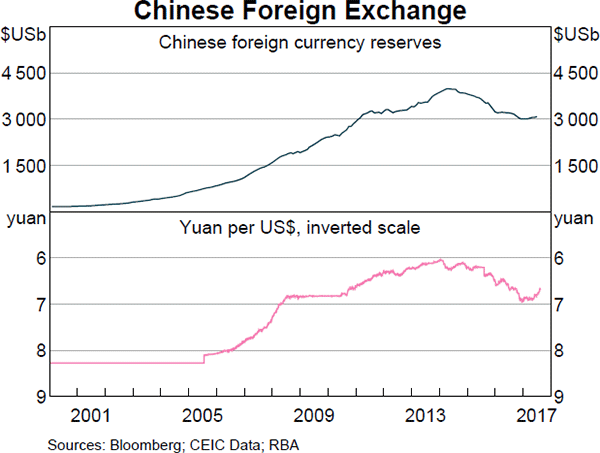# Fight Finance

#### CoursesTagsRandomAllRecentScores

For a price of $100, Andrea will sell you a 2 year bond paying annual coupons of 10% pa. The face value of the bond is$100. Other bonds with the same risk, maturity and coupon characteristics trade at a yield of 6% pa.

Would you like to the bond or politely ?

A method commonly seen in textbooks for calculating a levered firm's free cash flow (FFCF, or CFFA) is the following:

\begin{aligned} FFCF &= (Rev - COGS - Depr - FC - IntExp)(1-t_c) + \\ &\space\space\space+ Depr - CapEx -\Delta NWC + IntExp(1-t_c) \\ \end{aligned}
Does this annual FFCF or the annual interest tax shield?

The first payment of a constant perpetual annual cash flow is received at time 5. Let this cash flow be $C_5$ and the required return be $r$.

So there will be equal annual cash flows at time 5, 6, 7 and so on forever, and all of the cash flows will be equal so $C_5 = C_6 = C_7 = ...$

When the perpetuity formula is used to value this stream of cash flows, it will give a value (V) at time:

A stock will pay you a dividend of $10 tonight if you buy it today. Thereafter the annual dividend is expected to grow by 5% pa, so the next dividend after the$10 one tonight will be $10.50 in one year, then in two years it will be$11.025 and so on. The stock's required return is 10% pa.

What is the stock price today and what do you expect the stock price to be tomorrow, approximately?

The Australian cash rate is expected to be 2% pa over the next one year, while the Japanese cash rate is expected to be 0% pa, both given as nominal effective annual rates. The current exchange rate is 100 JPY per AUD.

What is the implied 1 year forward foreign exchange rate?

How much more can you borrow using an interest-only loan compared to a 25-year fully amortising loan if interest rates are 6% pa compounding per month and are not expected to change? If it makes it easier, assume that you can afford to pay \$2,000 per month on either loan. Express your answer as a proportional increase using the following formula:

$$\text{Proportional Increase} = \dfrac{V_\text{0,interest only}}{V_\text{0,fully amortising}} - 1$$

A company can invest funds in a five year project at LIBOR plus 50 basis points pa. The five-year swap rate is 4% pa. What fixed rate of interest can the company earn over the next five years by using the swap?

Which of the following interest rate quotes is NOT equivalent to a 10% effective annual rate of return? Assume that each year has 12 months, each month has 30 days, each day has 24 hours, each hour has 60 minutes and each minute has 60 seconds. APR stands for Annualised Percentage Rate.

A British man wants to calculate how many British pounds (GBP) he needs to buy a 1 million euro (EUR) apartment in Germany. The exchange rate is 1.42 USD per GBP and 1.23 USD per EUR. What is the EUR 1 million equivalent to in GBP?

The Chinese central bank has the largest amount of foreign currency reserves.What could the large amounts of foreign exchange reserves held by the Chinese government be used for in a currency crisis? China's currency is called the Renminbi (RMB) or Yuan (CNY). In a Chinese currency crisis the Chinese government is likely to use its FX reserves to: